# 1. Consider the following matrix and its reduced row echelon form [1 0 3 3 5...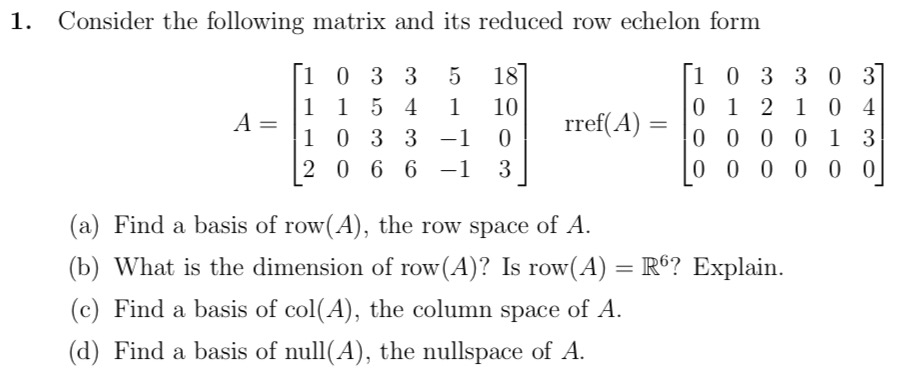1. Consider the following matrix and its reduced row echelon form [1 0 3 3 5 187 [1 0 3 3 0 37 1 1 5 4 1 10 0 1 2 1 0 - A=1 4 1 0 3 3 -1 0 rref(A) = 10 0 0 0 1 3 2 0 6 6 -1 3 | 0 0 0 0 0 0 (a) Find a basis of row(A), the row space of A. (b) What is the dimension of row(A)? Is row(A) = R6? Explain. (c) Find a basis of col(A), the column space of A. (d) Find a basis of null(A), the nullspace of A.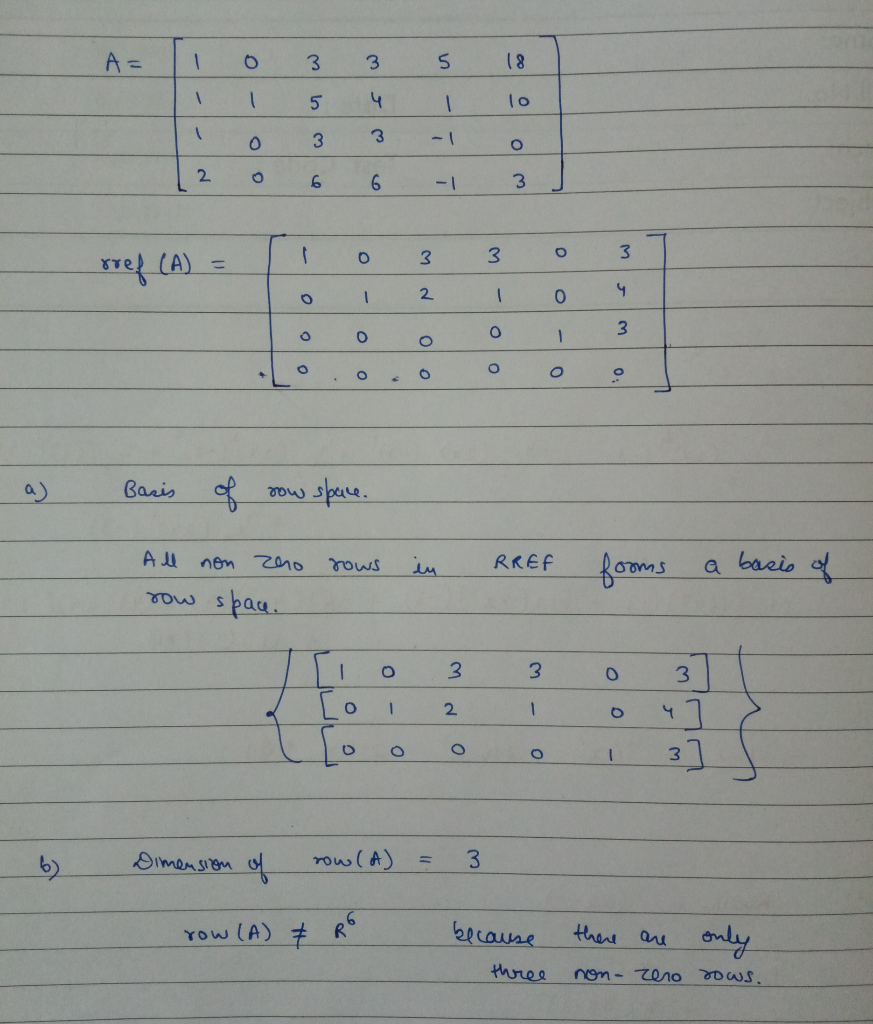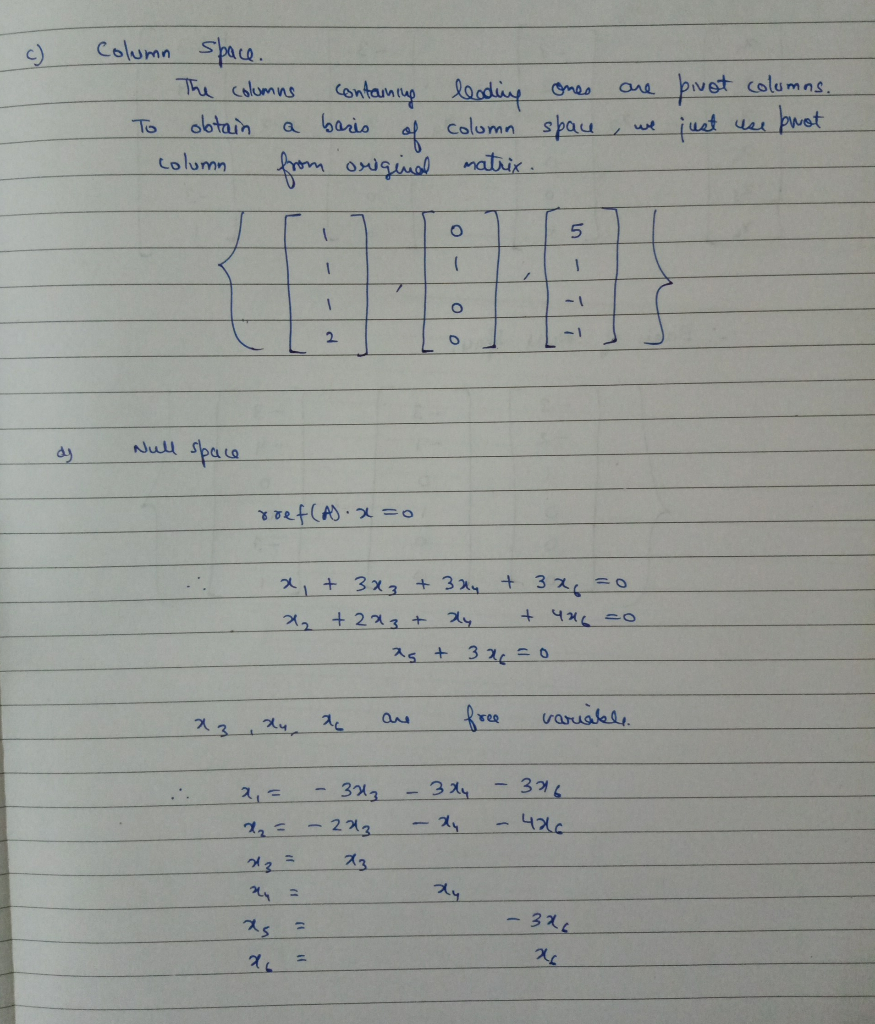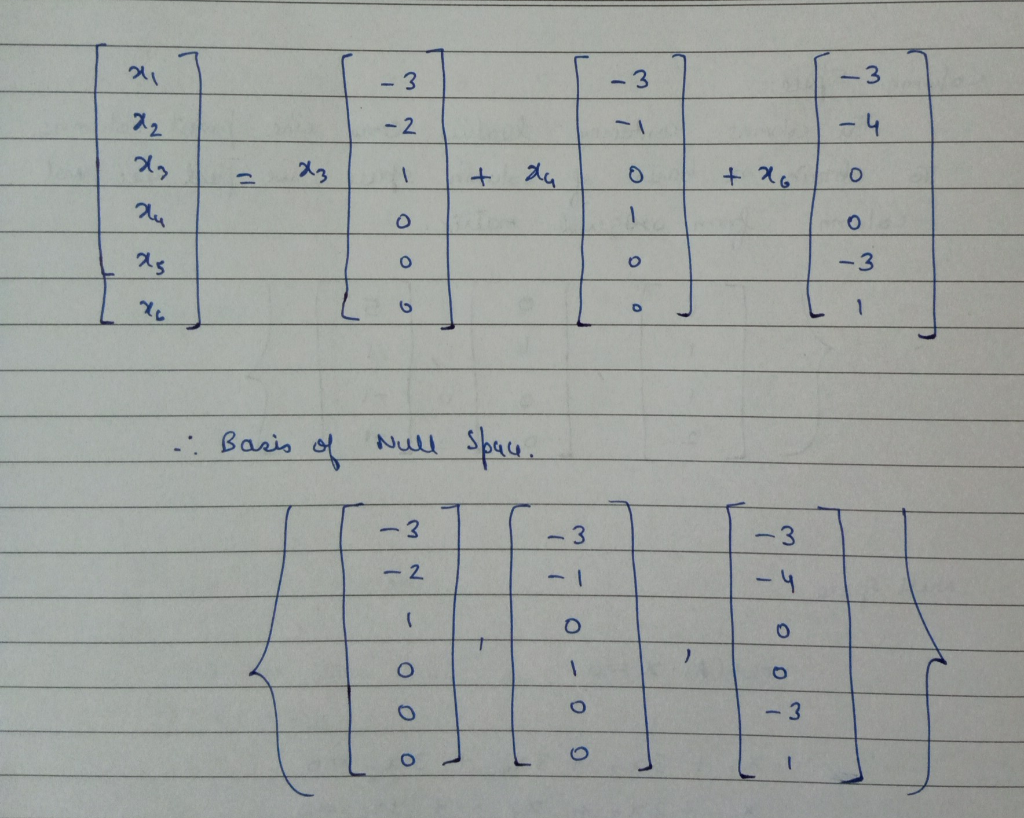#### Earn Coin

Coins can be redeemed for fabulous gifts.

Similar Homework Help Questions
• ### Question 3 [10 points] Consider the following matrix A and its reduced row-echelon form: A =...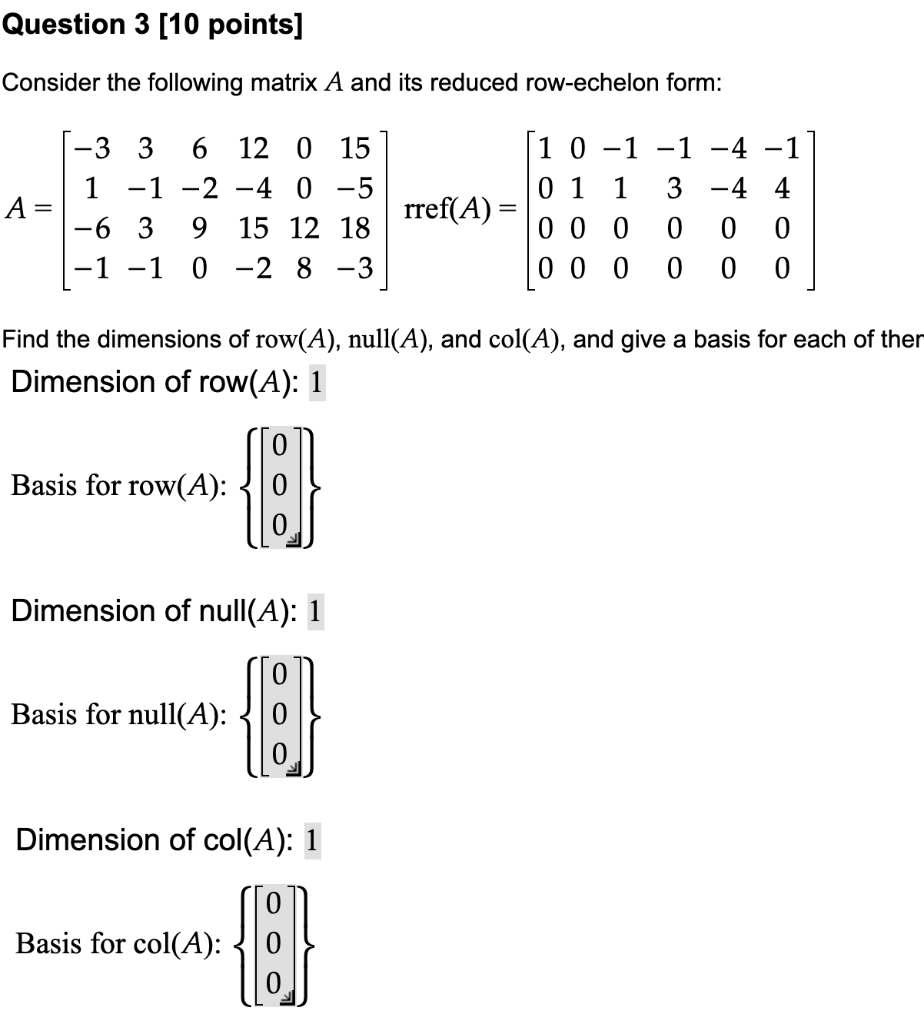Question 3 [10 points] Consider the following matrix A and its reduced row-echelon form: A = [-3 3 6 12 0 151 | 1 -1 -2 -4 0 -5 -6 3 9 15 12 18 rret(A) |-1 -1 0 -2 8 -3 [1 0 -1 -1 -4 -1] 01 1 3 -4 4 0 0 0 0 0 0 0 0 0 0 0 0 Find the dimensions of row(A), null(A), and col(A), and give a basis for each of...

• ### Question 3 please answer clearly. A matrix A and its reduced row echelon form are given...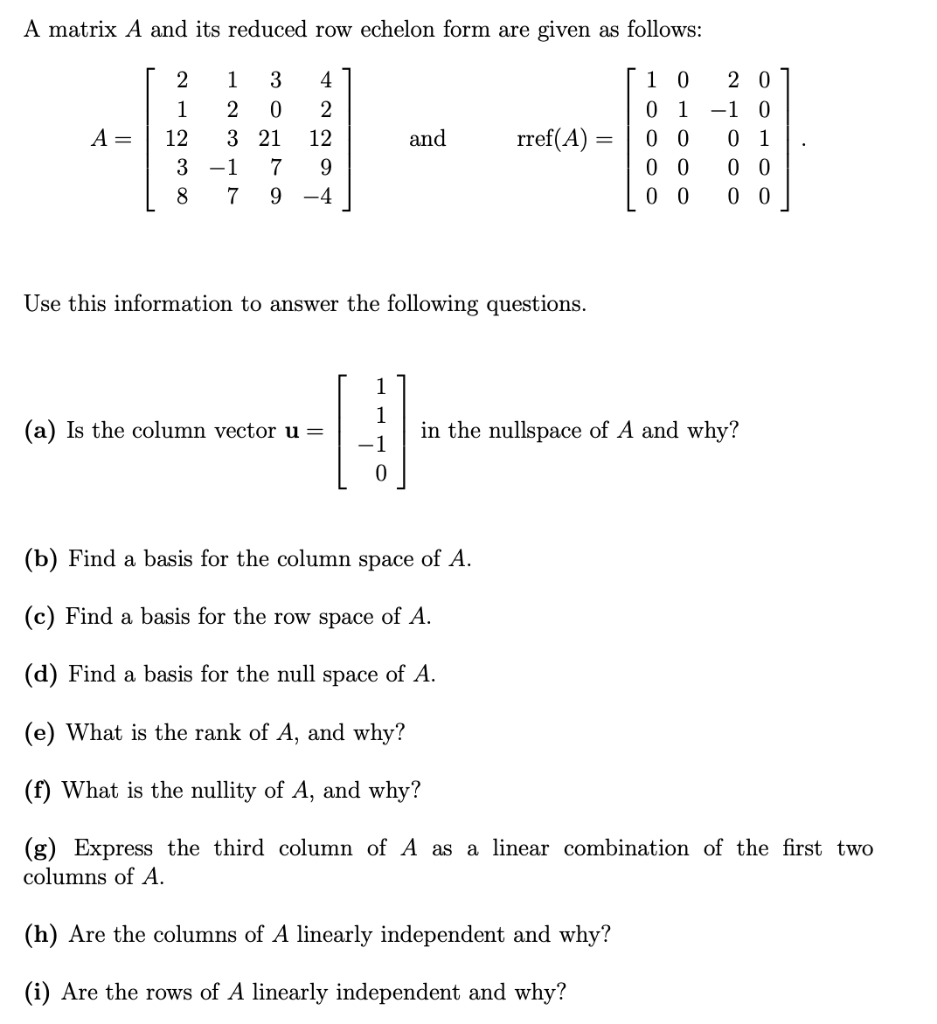Question 3 please answer clearly. A matrix A and its reduced row echelon form are given as follows: [ 2 1 3 41 | 1 2 0 2 A= 3 21 12 | 3 -1 7 9 18 7 9 -4 and rref(A) = [ 1 0 201 0 1 -1 0 0 0 0 1 0 0 0 0 | 0 0 0 0 Use this information to answer the following questions. (a) Is the column vector u= in...

• ### Given the matrix A and its reduced row echelon form R, answer the following questions. A=...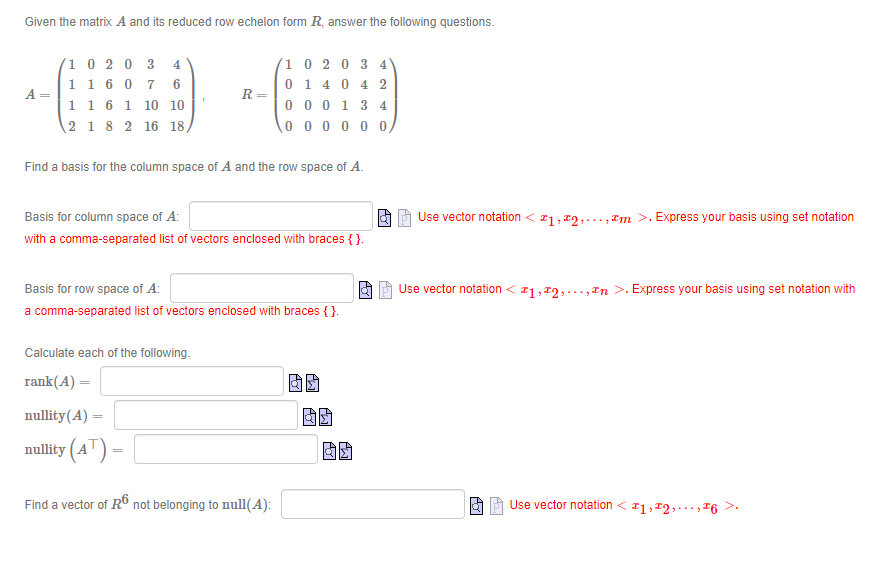Given the matrix A and its reduced row echelon form R, answer the following questions. A= 10 20 3 4 1 1 60 7 6 11 6 1 10 10 2 1 8 2 16 18 R= 1 0 2 0 3 4 0 14 0 4 2 000134 000000 Find a basis for the column space of A and the row space of A. Basis for column space of A: with a comma-separated list of vectors enclosed with braces...

• ### Given the matrix A and its reduced row echelon form R, answer the following questions. A=...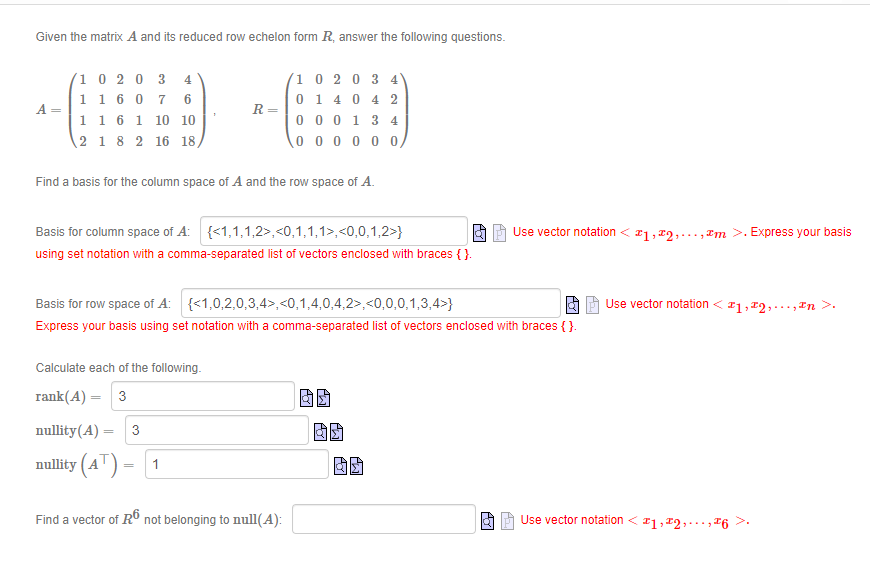Given the matrix A and its reduced row echelon form R, answer the following questions. A= 1 02 03 4 1 1 6 0 7 6 1 1 6 1 10 10 2 1 8 2 16 18 R= (10 20 3 4 01 4 0 4 2 000134 000000 Find a basis for the column space of A and the row space of A. Use vector notation <21,22,..., Im >. Express your basis Basis for column space of A:...

• ### Consider the following matrix A, and its reduced row-echelon form, B: A = 1 3 -1...

Consider the following matrix A, and its reduced row-echelon form, B: A = 1 3 -1 2 13 4 2 -4 4 2 2 5 -2 1 21 1 6 -15 28 B = ( row reduced) 1 0 -1 0 -2 0 1 0 0 5 0 0 0 1 0 0 0 0 0 0 (a) Write a basis for Nul(A)   (b) Write a basis for Col(A)

• ### Consider the matrix A and the reduced row echelon form of A. A 1 -2 -2...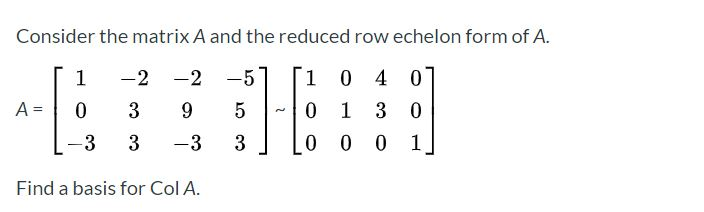Consider the matrix A and the reduced row echelon form of A. A 1 -2 -2 0 39 -3 3 -3 1 0 4 0 0 1 3 0 0 0 0 1 Find a basis for Col A.

• ### Use elementary row operations to reduce the given matrix to row echelon form and reduced row...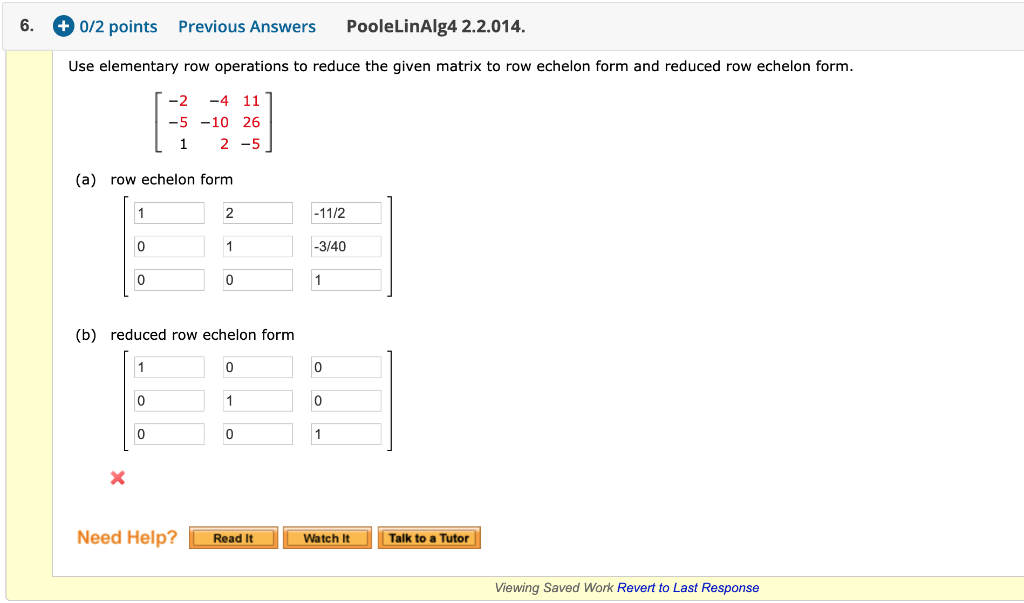Use elementary row operations to reduce the given matrix to row echelon form and reduced row echelon form. Please note when it hits REF and RREF. Thank you! 6. + 0/2 points Previous Answers PooleLinAlg4 2.2.014. Use elementary row operations to reduce the given matrix to row echelon form and reduced row echelon form. [-2 -4 11 | -5 -10 26 Li 2 -5] (a) row echelon form 2 1 -1172 -3/40 0 1 (b) reduced row echelon form 0...

• ### Given the matrix A and its reduced row echelon form R, answer the following questions. 4...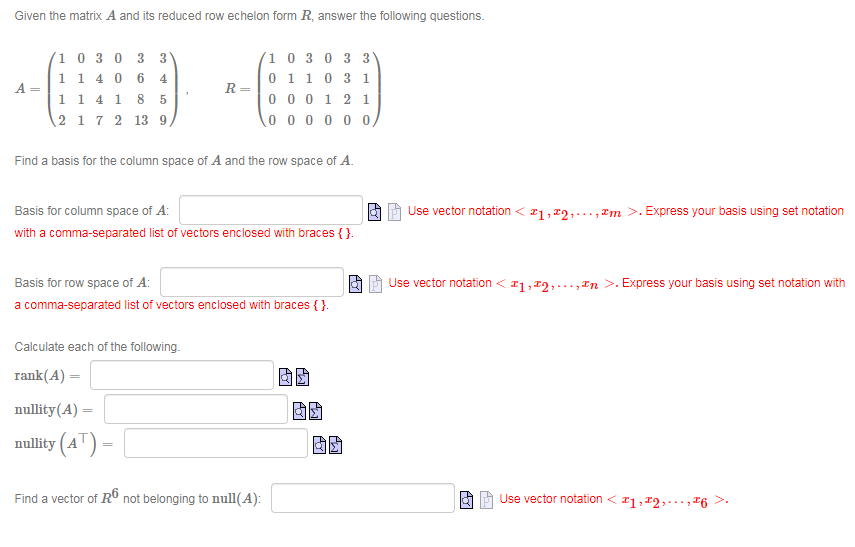Given the matrix A and its reduced row echelon form R, answer the following questions. 4 A 1 0 3 0 3 3 1 1406 1 1 4 1 8 2 1 7 2 13 9 R= 1 0 3 0 3 3 0110 31 000121 1000000 5 Find a basis for the column space of A and the row space of A. Ao Basis for column space of A: with a comma-separated list of vectors enclosed with braces {}....

• ### Consider the matrix A and the reduced row echelon form of A. 1 -2 -5 [1...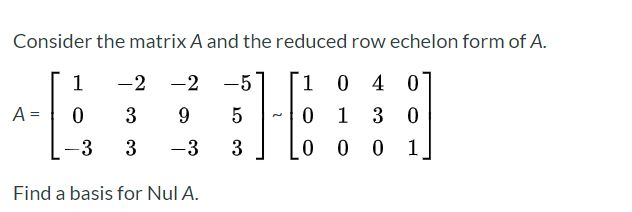Consider the matrix A and the reduced row echelon form of A. 1 -2 -5 [1 -2 9 0 4 0 1 3 0 A= 0 3 5 0 -3 3 -3 3 0 0 0 1 Find a basis for Nul A.

• ### 4. Consider the matrix 0- 3 1 -2 1 4 (a) Use Matlab to determine the reduced row echelon form of A (b) If v, V2, vs, v4...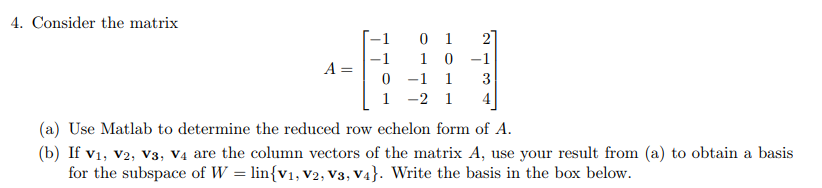4. Consider the matrix 0- 3 1 -2 1 4 (a) Use Matlab to determine the reduced row echelon form of A (b) If v, V2, vs, v4 are the column vectors of the matrix A, use your result from (a) to obtain a basis for the subspace of W-linsv1, V2, V3, V4. Write the basis in the box below 4. Consider the matrix 0- 3 1 -2 1 4 (a) Use Matlab to determine the reduced row echelon form...